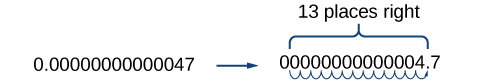# 1.2 Exponents and scientific notation  (Page 6/9)

 Page 6 / 9Be careful not to include the leading 0 in your count. We move the decimal point 13 places to the right, so the exponent of 10 is 13. The exponent is negative because we moved the decimal point to the right. This is what we should expect for a small number.

$4.7\text{\hspace{0.17em}}×\text{\hspace{0.17em}}{10}^{-13}$

## Scientific notation

A number is written in scientific notation    if it is written in the form $\text{\hspace{0.17em}}a\text{\hspace{0.17em}}×\text{\hspace{0.17em}}{10}^{n},$ where $\text{\hspace{0.17em}}1\le |a|<10\text{\hspace{0.17em}}$ and $\text{\hspace{0.17em}}n\text{\hspace{0.17em}}$ is an integer.

## Converting standard notation to scientific notation

Write each number in scientific notation.

1. Distance to Andromeda Galaxy from Earth: 24,000,000,000,000,000,000,000 m
2. Diameter of Andromeda Galaxy: 1,300,000,000,000,000,000,000 m
3. Number of stars in Andromeda Galaxy: 1,000,000,000,000
4. Diameter of electron: 0.00000000000094 m
5. Probability of being struck by lightning in any single year: 0.00000143

Write each number in scientific notation.

1. U.S. national debt per taxpayer (April 2014): $152,000 2. World population (April 2014): 7,158,000,000 3. World gross national income (April 2014):$85,500,000,000,000
4. Time for light to travel 1 m: 0.00000000334 s
5. Probability of winning lottery (match 6 of 49 possible numbers): 0.0000000715
1. $1.52×{10}^{5}$
2. $7.158×{10}^{9}$
3. $8.55×{10}^{13}$
4. $3.34×{10}^{-9}$
5. $7.15×{10}^{-8}$

## Converting from scientific to standard notation

To convert a number in scientific notation    to standard notation, simply reverse the process. Move the decimal $\text{\hspace{0.17em}}n\text{\hspace{0.17em}}$ places to the right if $\text{\hspace{0.17em}}n\text{\hspace{0.17em}}$ is positive or $\text{\hspace{0.17em}}n\text{\hspace{0.17em}}$ places to the left if $\text{\hspace{0.17em}}n\text{\hspace{0.17em}}$ is negative and add zeros as needed. Remember, if $\text{\hspace{0.17em}}n\text{\hspace{0.17em}}$ is positive, the value of the number is greater than 1, and if $\text{\hspace{0.17em}}n\text{\hspace{0.17em}}$ is negative, the value of the number is less than one.

## Converting scientific notation to standard notation

Convert each number in scientific notation to standard notation.

1. $3.547\text{\hspace{0.17em}}×\text{\hspace{0.17em}}{10}^{14}$
2. $-2\text{\hspace{0.17em}}×\text{\hspace{0.17em}}{10}^{6}$
3. $7.91\text{\hspace{0.17em}}×\text{\hspace{0.17em}}{10}^{-7}$
4. $-8.05\text{\hspace{0.17em}}×\text{\hspace{0.17em}}{10}^{-12}$

Convert each number in scientific notation to standard notation.

1. $7.03\text{\hspace{0.17em}}×\text{\hspace{0.17em}}{10}^{5}$
2. $-8.16\text{\hspace{0.17em}}×\text{\hspace{0.17em}}{10}^{11}$
3. $-3.9\text{\hspace{0.17em}}×\text{\hspace{0.17em}}{10}^{-13}$
4. $8\text{\hspace{0.17em}}×\text{\hspace{0.17em}}{10}^{-6}$
1. $703,000$
2. $-816,000,000,000$
3. $-0.000\text{\hspace{0.17em}}000\text{\hspace{0.17em}}000\text{\hspace{0.17em}}000\text{\hspace{0.17em}}39$
4. $0.000008$

## Using scientific notation in applications

Scientific notation, used with the rules of exponents, makes calculating with large or small numbers much easier than doing so using standard notation. For example, suppose we are asked to calculate the number of atoms in 1 L of water. Each water molecule contains 3 atoms (2 hydrogen and 1 oxygen). The average drop of water contains around $\text{\hspace{0.17em}}1.32\text{\hspace{0.17em}}×\text{\hspace{0.17em}}{10}^{21}\text{\hspace{0.17em}}$ molecules of water and 1 L of water holds about $\text{\hspace{0.17em}}1.22\text{\hspace{0.17em}}×\text{\hspace{0.17em}}{10}^{4}\text{\hspace{0.17em}}$ average drops. Therefore, there are approximately $\text{\hspace{0.17em}}3\cdot \left(1.32\text{\hspace{0.17em}}×\text{\hspace{0.17em}}{10}^{21}\right)\cdot \left(1.22\text{\hspace{0.17em}}×\text{\hspace{0.17em}}{10}^{4}\right)\approx 4.83\text{\hspace{0.17em}}×\text{\hspace{0.17em}}{10}^{25}\text{\hspace{0.17em}}$ atoms in 1 L of water. We simply multiply the decimal terms and add the exponents. Imagine having to perform the calculation without using scientific notation!

#### Questions & Answers

if sin15°=√p, express the following in terms of p
Norman Reply
prove sin²x+cos²x=3+cos4x
Kiddy Reply
the difference between two signed numbers is -8.if the minued is 5,what is the subtrahend
jeramie Reply
the difference between two signed numbers is -8.if the minuend is 5.what is the subtrahend
jeramie
what are odd numbers
micheal Reply
numbers that leave a remainder when divided by 2
Thorben
1,3,5,7,... 99,...867
Thorben
7%2=1, 679%2=1, 866245%2=1
Thorben
the third and the seventh terms of a G.P are 81 and 16, find the first and fifth terms.
Suleiman Reply
if a=3, b =4 and c=5 find the six trigonometric value sin
Martin Reply
ask
Ans
pls how do I factorize x⁴+x³-7x²-x+6=0
Gift Reply
in a function the input value is called
Rimsha Reply
how do I test for values on the number line
Modesta Reply
if a=4 b=4 then a+b=
Rimsha Reply
a+b+2ab
Kin
commulative principle
DIOSDADO
a+b= 4+4=8
Mimi
If a=4 and b=4 then we add the value of a and b i.e a+b=4+4=8.
Tariq
what are examples of natural number
sani Reply
an equation for the line that goes through the point (-1,12) and has a slope of 2,3
Katheryn Reply
3y=-9x+25
Ishaq
show that the set of natural numberdoes not from agroup with addition or multiplication butit forms aseni group with respect toaaddition as well as multiplication
Komal Reply
x^20+x^15+x^10+x^5/x^2+1
Urmila Reply
evaluate each algebraic expression. 2x+×_2 if ×=5
Sarch Reply

### Read also:

#### Get Jobilize Job Search Mobile App in your pocket Now!

Source:  OpenStax, Algebra and trigonometry. OpenStax CNX. Nov 14, 2016 Download for free at https://legacy.cnx.org/content/col11758/1.6
Google Play and the Google Play logo are trademarks of Google Inc.

Notification Switch

Would you like to follow the 'Algebra and trigonometry' conversation and receive update notifications?ByByBy Anonymous UserBy Janet ForresterBy OpenStaxBy Jesenia WoffordBy Jessica CollettBy OpenStaxBy Katherina jennife...By RhodesBy Megan EarhartBy Ryan Lowe